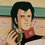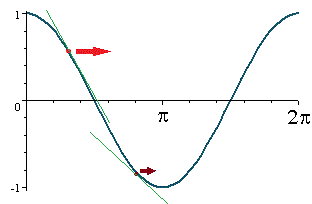# Show why the value converges to $\pi$

$a_0=1$

$a_{n+1}=a_n+\sin{(a_n)}$

Explain why the following occurs:

$a_0=1$

$a_1=1+\sin{(1)}\approx 1.841470985$

$a_2=1+\sin{(1)}+\sin{(1+\sin{(1)})}\approx 2.805061709$

$a_3=1+\sin{(1)}+\sin{(1+\sin{(1)})}+\sin{(1+\sin{(1)}+\sin{(1+\sin{(1)})})}\approx 3.135276333$

$a_4\approx 3.141592612$

$a_5\approx 3.141592654\approx\pi$Note by Jack Han
6 years, 4 months ago

This discussion board is a place to discuss our Daily Challenges and the math and science related to those challenges. Explanations are more than just a solution — they should explain the steps and thinking strategies that you used to obtain the solution. Comments should further the discussion of math and science.

When posting on Brilliant:

• Use the emojis to react to an explanation, whether you're congratulating a job well done , or just really confused .
• Ask specific questions about the challenge or the steps in somebody's explanation. Well-posed questions can add a lot to the discussion, but posting "I don't understand!" doesn't help anyone.
• Try to contribute something new to the discussion, whether it is an extension, generalization or other idea related to the challenge.

MarkdownAppears as
*italics* or _italics_ italics
**bold** or __bold__ bold
- bulleted- list
• bulleted
• list
1. numbered2. list
1. numbered
2. list
Note: you must add a full line of space before and after lists for them to show up correctly
paragraph 1paragraph 2

paragraph 1

paragraph 2

[example link](https://brilliant.org)example link
> This is a quote
This is a quote
    # I indented these lines
# 4 spaces, and now they show
# up as a code block.

print "hello world"
# I indented these lines
# 4 spaces, and now they show
# up as a code block.

print "hello world"
MathAppears as
Remember to wrap math in $$ ... $$ or $ ... $ to ensure proper formatting.
2 \times 3 $2 \times 3$
2^{34} $2^{34}$
a_{i-1} $a_{i-1}$
\frac{2}{3} $\frac{2}{3}$
\sqrt{2} $\sqrt{2}$
\sum_{i=1}^3 $\sum_{i=1}^3$
\sin \theta $\sin \theta$
\boxed{123} $\boxed{123}$

Sort by:

Let's see here...

The interesting thing I was talking about is the fact that the given series always converges into a value $a_n$ such that $sin(a_n)=0$ for different values of $a_0$. To put it in more exact terms, it always converges to a value of $a_n$ such that $cos(a_n)=-1\Rightarrow a_n=m\pi$, where $m$ is an odd integer. ($m$ can also be even, but that is a degenerate case where all terms are same)

I'm going to use something here that I actually learned from gradient descent. If you don't know what it is, you can google it. But, the mathematics used below is an extremely tame form and is easy to understand with little knowledge of calculus.

Consider the function $f\left( x \right) =\cos { (x) } \\ \Rightarrow \frac { df\left( x \right) }{ dx } =-\sin { (x) }$

Now see what happens when we take some arbitrary value of $x$ (say $x=1$)and then do the following repeatedly:

$x:=x-\frac { df\left( x \right) }{ dx }=x+\sin { (x) }$ (":=" is the assignment operator )In the above figure, we can see two points marked. One is red, which represents the first value of $x$($=1$). The other is brown, and is after one iteration of above step.

We can see that when we do $x:=x+sin(x)$, what is actually happening is that $x$ is surfing along the slope of the curve $cos(x)$. We move the value of $x$ down the tangent. Change $x$ little by little, so that finally, after many iterations it moves closer and closer to the minima, i.e $x=\pi$.

I know this is not a definitive proof of what happens... I'm sure you will realize the importance of this once you understand what is happening.

In general, series defined as $a_n=a_{n-1}-\alpha\frac { df\left(a_{n-1} \right) }{ da_{n-1} }$

Will converge to the nearest value of $a$ (nearest to $a_0$) such that $f(a)$ is minimum, provided the value of $\alpha$ is not too large.

- 6 years, 4 months ago

Yes, that was awesome, that is basically the newton Rhapsody method of estimation of roots, doing the following iteration for any curve will eventually lead us to the nearest root, that is great, actually i think this is pretty much the solution +1

- 6 years, 4 months ago

Good work! Newton's method for estimating roots.

Pretty much seals the deal. Great solution +1.

- 6 years, 4 months ago

Good work.

- 6 years, 4 months ago

- 6 years, 4 months ago

As usual, since the series converges.. this means that when $n\to\infty$ ,

$a_{n+1}=a_{n}$. But $a_{n+1}=a_{n}+sin(a_{n})$

$\Rightarrow sin(a_{n})=0$

Now how do we know that $a_{n}=\pi$? We know this since $a_0=1$ and the series is constantly increasing. Therefore, it converges onto the first value of $x>1$ such that $sin(x)=0$.

- 6 years, 4 months ago

Bro , But It is not always true $\lim _{ n\rightarrow \infty }{ ({ a }_{ n }) } =\lim _{ n\rightarrow \infty }{ { (a }_{ n+1 }) }$ .

- 6 years, 4 months ago

Why not? Do you have a counter-example?

- 6 years, 4 months ago

Which value converges to pi ??

- 6 years, 4 months ago

If you call L the value of the limit you obtain sin(L)=0. Now L can be pi or zero but zero is impossible because of the initial condition. More precisely you can say that the value of the sequence is LOW bounded

- 6 years, 4 months ago

This is turning out to be very interesting...

I want to know if we can find a general form for a function $f(x)$ such that the series $a_1,a_2,...$ defined by:

$a_{n+1}=a_{n-1}+f(a_{n-1})$

Converges for a given value of $a_0$.

Further, is it true that all of the values of such $a_n$ as $n\to \infty$ satisfy $f(a_n)=0$?

- 6 years, 4 months ago

I think the answer to this is going to be extremely interesting.... I have a feeling... Is anyone else thinking what I'm thinking?

- 6 years, 4 months ago

What are you thinking?

- 6 years, 4 months ago